## Spring Design

Design information for engineers, such as spring calculation formulas,

which are the basis of spring design, can be found here.

### Meaning of Symbols

The symbols and units used for spring design are shown in Table 1. The values of the longitudinal elastic modulus (E) are shown in Table 2.

Table 1. Symbols and units used in calculations

Symbol Meaning of Symbol Unit
D Outer diameter mm
d Inner diameter mm
t Thickness mm
H0 Free height mm
h0 Total deflection（H0-t） mm
E Longitudinal elastic modulus of material
(E=2.06×105
N/mm2
v Poisson’s ratio of material（0.3)
P Load applied N
δ Deflection of a single disc spring mm
σI Stress at position I N/mm2
σII Stress at position II N/mm2
σIII Stress at position III N/mm2
σIV Stress at Position IV N/mm2
PG Load of the spring stack N
δG Deflection of the spring stack mm
n Number of disc springs stacked in parallel Piece
m Number of sets stacked in series Set
L0 Free height of the spring stack mm
R Chamfered part’s radius mm

Table 2.　Longitudinal elastic modulus：E（N/m㎡）

Material E Value
Spring steel material 206×103
Stainless steel SUS302

SUS304

SUS631

186×103
186×103
196×103

Note that the chamfered amount of corner II is equal to or more than R of corner I and III.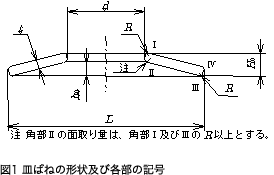### Coefficients Used for Calculation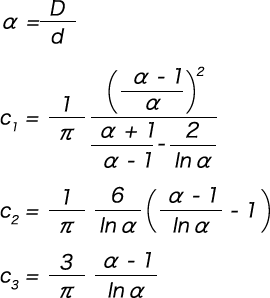### Calculation of Load

For the load (P), add the expression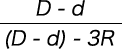that takes into consideration the chamfer of corner R, and calculate as per the following formula.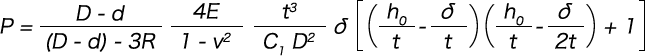### Calculation of Stress

Calculate the stress at each corner of positions I, II, III, and IV shown in Figure 2. When the value is positive, it is tensile stress. When the value is negative, it is compressive stress.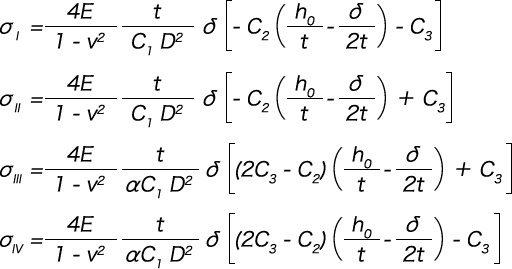### Corner Position of Maximum Stress

When examining the fatigue life, we need to examine the repeatability at the position where the maximum stress occurs from among the stress that occurs at the corners. The maximum stress position of the disc spring differs depending on the ratio of the outer and inner diameters and the ratio of the total amount of deflection to the thickness. The position where the maximum tensile stress is generated from these relationships is shown in Figure 2, therefore we can examine the maximum stress using this figure as reference.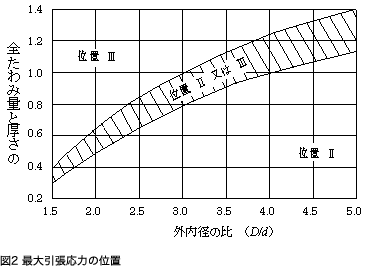### Stacking Disc Springs

Disc springs can obtain various load characteristics depending on how they are stacked.

#### Method of Stacking: In Parallel and In Series

In Parallel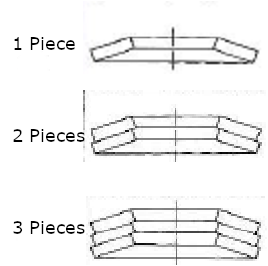Figure 3. Parallel Stacking

・Load: Increases

・ Deflection: No change

Load: Load per single spring × No. of springs in parallel

Springs stacked in the same direction as shown in the figure.

In Series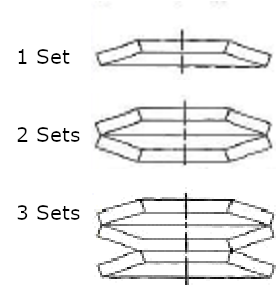Figure 3. Serial Stacking

・Load: No change

・Deflection: Increases

Deflection：Deflection amount per disc spring × No. of springs in series

Springs stacked alternately as shown in the figure.

#### Change in Load and Deflection due to Parallel / Series Stacking

As shown in the figures, the load and deflection change depending on whether the springs are stacked in parallel or series.Figure 5. Changes in Load and Deflection Due to Parallel / Series Stacking

#### Difference between Calculated Values and Measured Values

The measured load curve is lower than the calculated load curve due to the effect of initial strain and so forth up to 40% of the total deflection, and the load increases rapidly when it exceeds 75% of the total deflection. It is recommended to try one’s best to use the spring within the range where the load characteristics are stable. The DIN standard specifies the maximum deflection for use as δ = 0.75 h (total deflection amount is δ = 0.75 h).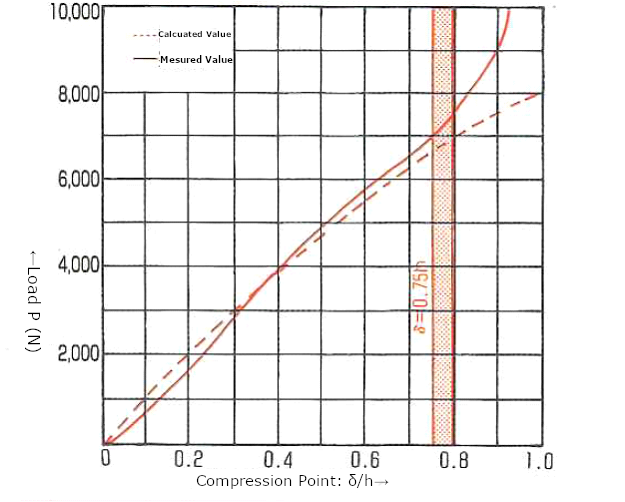Figure 6. Difference between Calculated and Measured Values

#### Guides and Clearance

When using disc springs in combination, a guide (shaft, case, etc.) is required on the inner or outer diameter side. The shape of the spring changes due to compression, and so it is important to consider clearance with a guide.

Example: Change in diameter during compression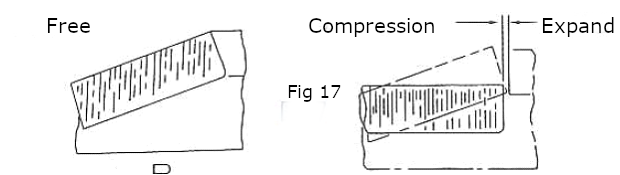Figure 7. Change in Diameter When Disc Spring is Compressed

The inner diameter increases and the outer diameter decreases as the spring is compressed.

The table below shows the general clearance for disc springs. (As it is an in-house standard, it differs from the JIS B 2706 standard.)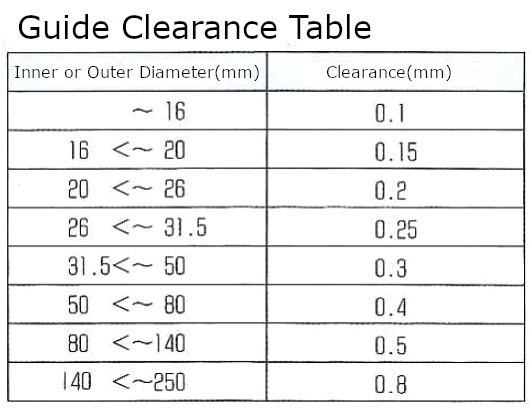Table 3. Disc Spring Guides and Clearances

※The clearances in the table are the values for one side of the diameter. The clearances for both ends (twice the notation) are required in actual design.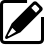Product information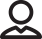Our Proposals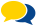Popular questions